## Area and Perimeter of Rectangles and Squares

#### What is the perimeter of a Rectangle?

The perimeter of a rectangle is the length of all its 4 sides. Formula to calculate the perimeter of a rectangle is:

Perimeter of Rectangle  =  2  ×  Length  +  2  ×  Breadth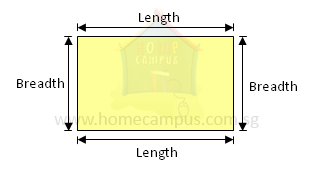The perimeter can be represented using a model as below.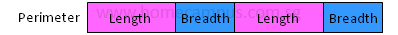=  2  ×  Length  +  2  ×  Breadth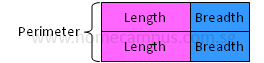Length  +  Breadth  =  Perimeter  ÷  2

#### What is the perimeter of a Square?

Perimeter of Square  =  4  ×  Length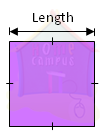Property of a square: All sides are equal.
Perimeter  =  Length  +  Length  +  Length  +  Length
=  4  ×  Length

#### What is the formula to calculate Area of a Rectangle and a Square?

Area of Rectangle  =  Length  ×  Breadth

Area of Square  =  Length  ×  Length

Let's find the area of the rectangle below.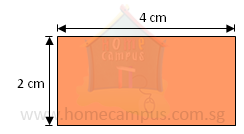To find the area of the rectangle, we find out how many one-centimetre squares we can fit into the rectangle.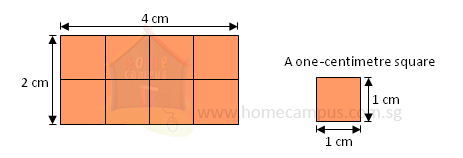Area of the rectangle  =  4  ×  2
=  8 cm2

We need 8 one-centimetre squares to make a rectangle 4 cm long and 2 cm wide.
The area of the rectangle is 8 cm2.

#### 1. Find the area and perimeter of a rectangle with a length of 6 cm and a breadth of 4 cm.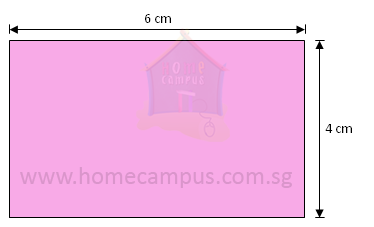=   6  ×  4

=   24 cm2

Perimeter  =  2  ×  Length  +  2  ×  Breadth

=   2  ×  6  +  2  ×  4

=   12  +  8

=   20 cm

#### 2. A square table cloth has a side of 5 m. What are its perimeter and area?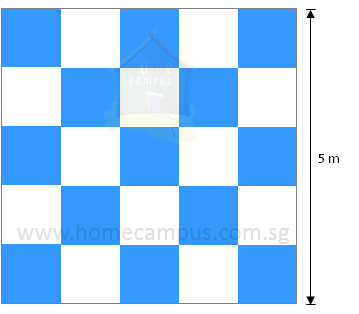Area  =  5  ×  5

=   25 m2

Perimeter  =  4  ×  5
=   20 m

#### 3. A 4 m long rectangular mat has an area of 12 m2. a)  What is its breadth? b)  What is its perimeter?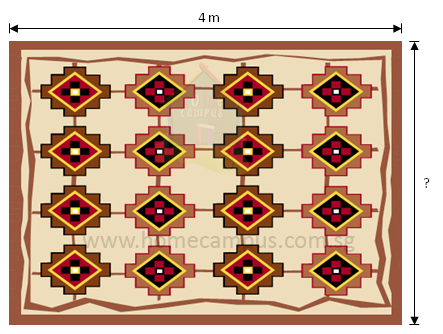a)   Area  =  Length  ×  Breadth

=   12  ÷  4

=   3 m

b)   Perimeter  =  2  ×  Length  +  2  ×  Breadth

=   ( 2  ×  4 )  +  ( 2  ×  3 )

=   8  +  6

=   14 m

#### 4. Monty joins 4 identical straws to form a square of area 144 cm2. What is the perimeter of the square formed?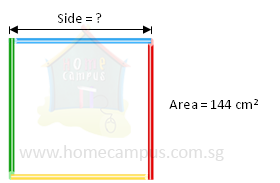Area of Square  =  Side  ×  Side

144  =  12  ×  12

Side  =  12 cm

Perimeter  =  4  ×  Side

=   4  ×  12

=   48 cm

#### 5. The figure below is made of Rectangle A and Square B. Find the area and perimeter of the figure.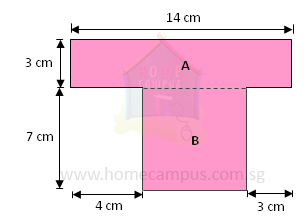Area of Rectangle A  =  14  ×  3

=   42 cm2

Area of Square B  =  7  ×  7

=   49 cm2

Area of Figure  =  42  +  49

=   91 cm2

Perimeter of Figure  =  14  +  3  +  4  +  7  +  7  +  7  +  3  +  3

=   48 cm

#### 6. All lines in the figure below meet at right angles. Find the area and perimeter of the figure.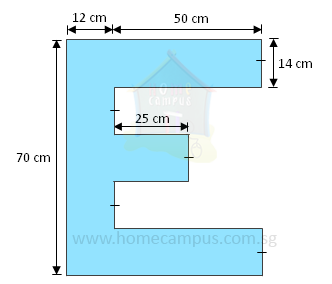Perimeter of Figure

=   12 + 50 + 14 + 50 + 14 + 25 + 14 + 25 + 14 + 50 + 14 + 50 + 12 + 70

=   414 cm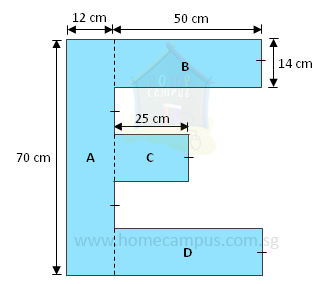Area of Figure  =  Area of A  +  Area of B  +  Area of C  +  Area of D

=   (70 × 12)  +  (50 × 14)  +  (25 × 14)  +  (50 × 14)

=   840  +  700  +  350  +  700

=   2590 cm2

#### 7. Carlos mounts his painting on a frame as shown below. a)   Find the perimeter of the painting. b)   Find the area of the painting. c)   Find the area of the border of the frame.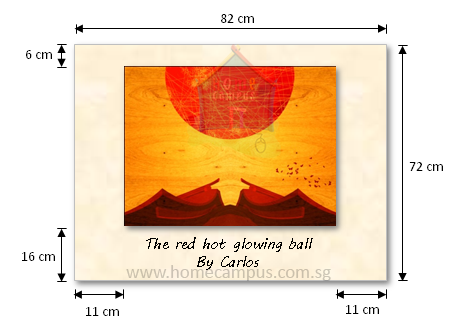a) Length of painting  =  82 cm  −  11 cm  −  11 cm

=   60 cm

Breadth of painting  =  72 cm  −  6 cm  −  16 cm

=   50 cm

Perimeter of painting  =  2  ×  Length of painting  +  2  ×  Breadth of painting

=   (2 × 60 cm)  +  (2 × 50 cm)

=   120 cm  +  100 cm

=   220 cm

b) Area of painting  =  Length of painting  ×  Breadth of painting

=   60 cm  ×  50 cm

=   3000 cm2

c) Area of frame  =  82 cm  ×  72 cm

=   5904 cm2

Area of border  =  Area of frame  −  Area of painting

=   5904  −  3000

=   2904 cm2

#### 8. The figure below shows wooden tiling around a swimming pool. a)   Find the perimeter of the figure. b)   Find the area of the pool. c)   Find the area of the tiling.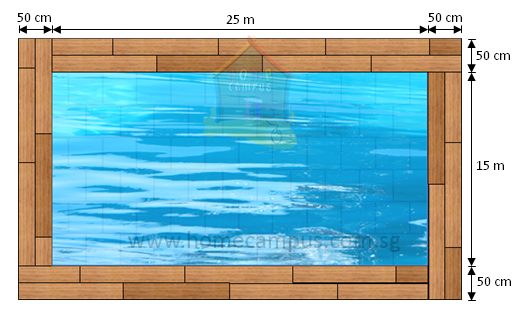a) Length of big rectangle  =  50 cm  +  25 m  +  50 cm

=   100 cm  +  25 m

=   1 m  +  25 m

=   26 m

Breadth of big rectangle  =  50 cm  +  15 m  +  50 cm

=   100 cm  +  15 m

=   1 m  +  15 m

=   16 m

Perimeter of figure  =  Perimeter of big rectangle

=   (2 × 26 m)  −  (2 × 16 m)

=   52 m  −  32 m

=   20 m
100 cm = 1 m
b) Area of pool  =  Area of small rectangle

=   25 m  ×  15 m

=   375 m2

c) Area of tiling  =  Area of big rectangle  −  Area of small rectangle

=   (26 m  ×  16 m)  −  375 m2

=   416 m2  −  375 m2

=   41 m2